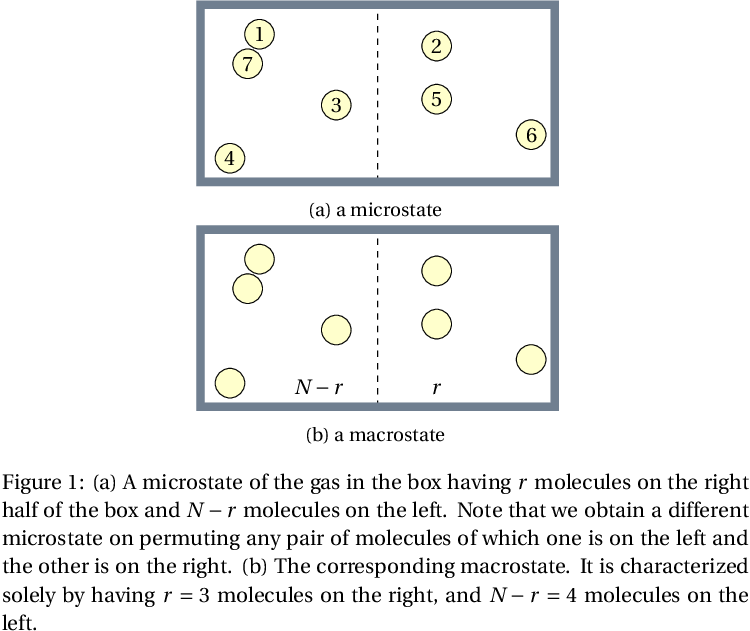# The subcaption Package

Documentation figures The subcaption package allows you to add separate captions to parts of a multipart figure or table. It supersedes older packages such as subfig and subfigure. \usepackage{subcaption} % Use subcaption to add separate captions to parts of a multipart fig/table

The example below shows how to create a figure with two subfigures. Each subfigure is drawn by tikz, but you can also use \includegraphics[options]{filename}

\begin{figure}[tb]
\centering
\subcaptionbox{a microstate\label{fig:amicrostate}}{
\begin{tikzpicture}[scale=1]
\def\x{3}
\def\y{3}
\draw[dashed] (0,0) -- (0,\y);
\draw[line width=4pt,color=SlateGray] (-\x,0) rectangle (\x,\y);
\foreach \x/\y/\n in {-2/2.5/1, 1/2.3/2, -0.7/1.3/3,
-2.5/0.4/4, 1/1.4/5, 2.6/0.8/6, -2.2/2/7} {
\node[draw,circle,inner sep=2pt,fill=yellow!20] at (\x,\y) {\n};
}
\end{tikzpicture}
}
\subcaptionbox{a macrostate\label{fig:amacrostate}}{
\begin{tikzpicture}[scale=1]
\def\x{3}
\def\y{3}
\draw[dashed] (0,0) -- (0,\y);
\draw[line width=4pt,color=SlateGray] (-\x,0) rectangle (\x,\y);
\foreach \x/\y/\n in {-2/2.5/1, 1/2.3/2, -0.7/1.3/3,
-2.5/0.4/4, 1/1.4/5, 2.6/0.8/6, -2.2/2/7} {
\node[draw,circle,inner sep=2pt,fill=yellow!20] at (\x,\y) {\phantom{\n}};
}
\node[anchor=south] at (-\x/3,0.1) {$N-r$};
\node[anchor=south] at (\x/3,0.1) {$\vphantom{N}r$};
\end{tikzpicture}
}
\caption[Microstates and macrostates of a gas]{
(a) A microstate of the gas in the box having $r$ molecules on
the right half of the box and $N-r$ molecules on the left. Note that
we obtain a different microstate on permuting any pair of molecules
of which one is on the left and the other is on the right. (b) The
corresponding macrostate. It is characterized solely by having $r = 3$ molecules on the right, and $N-r=4$ molecules on the left.
}
\label{fig:boltzbeetleA}
\end{figure}



By default, the letter of the subfigure is tacked onto the figure number (Fig. 3a). If you want the subfigure designation to be set off in parentheses (Fig. 3(a)), load the package with the following code:

\usepackage[labelformat=simple]{subcaption}
\renewcommand\thesubfigure{(\alph{subfigure})}